# 1. Let B1 = {1, æ, } and B2 = {x - 1,22 - 1 -...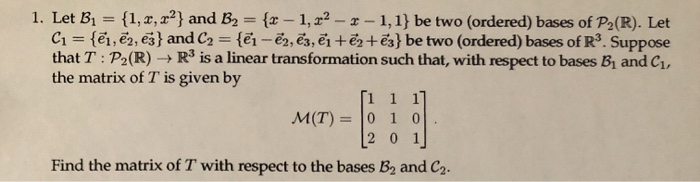1. Let B1 = {1, æ, } and B2 = {x - 1,22 - 1 - 1,1} be two (ordered) bases of P2(R). Let C1 = {ēm, ēm, ēj} and C2 = {@i - ēm, ēz, ?i + ?2+ēz} be two (ordered) bases of R3. Suppose the matrix of T is given by [1 1 1] [2 0 1 Find the matrix of T with respect to the bases B, and C.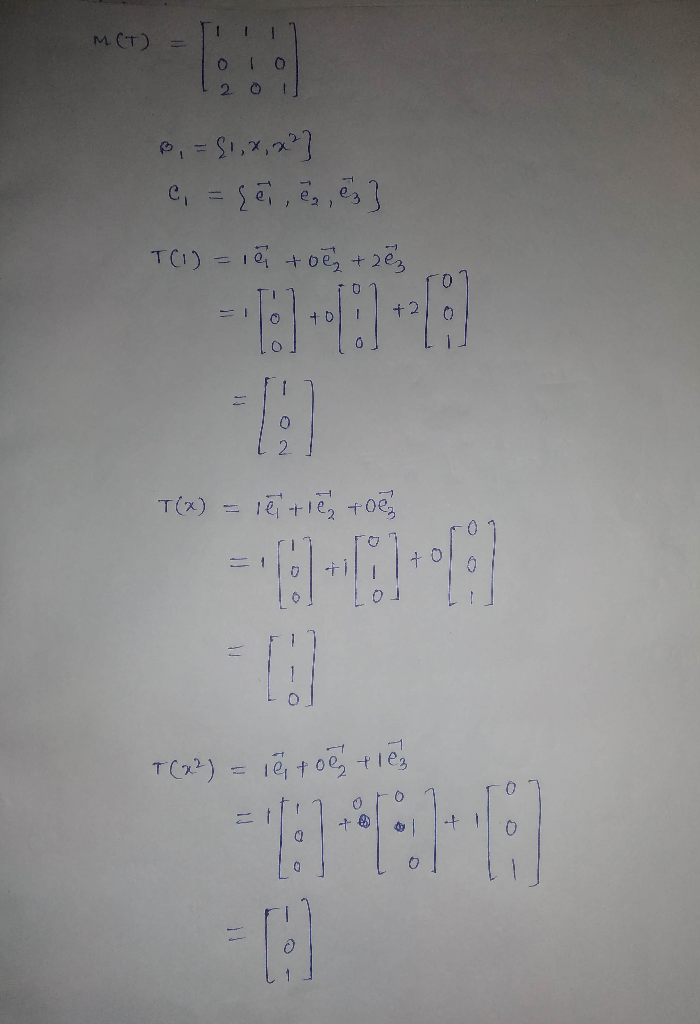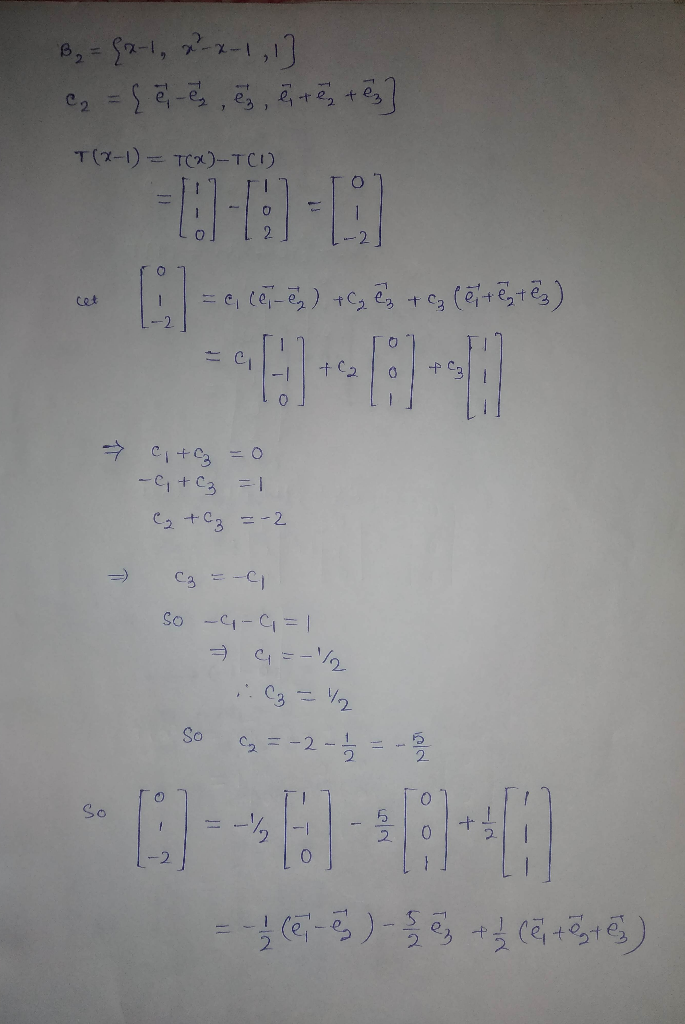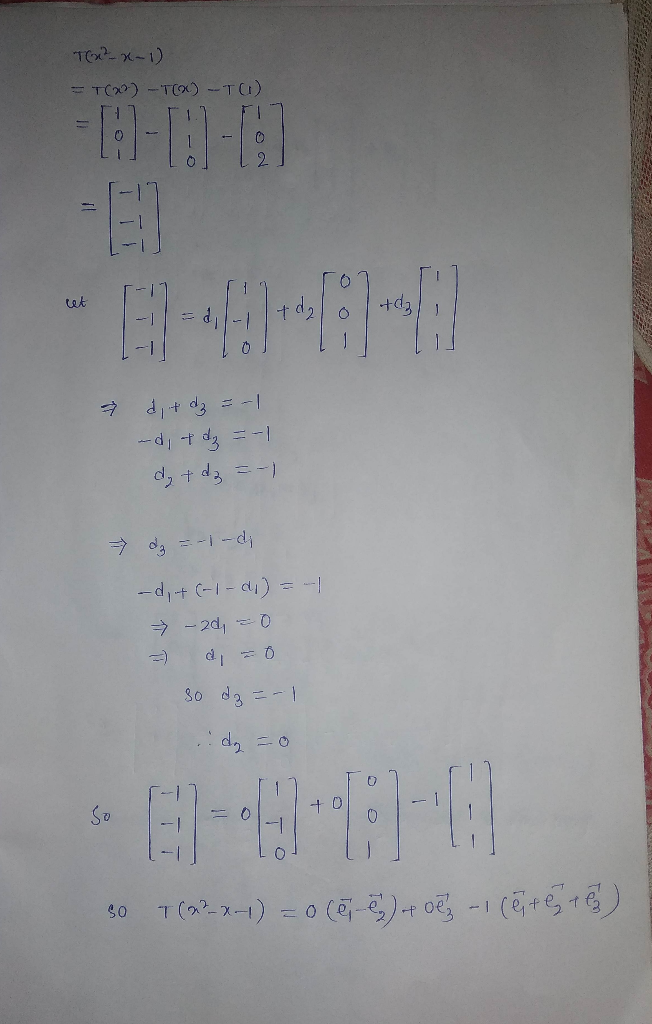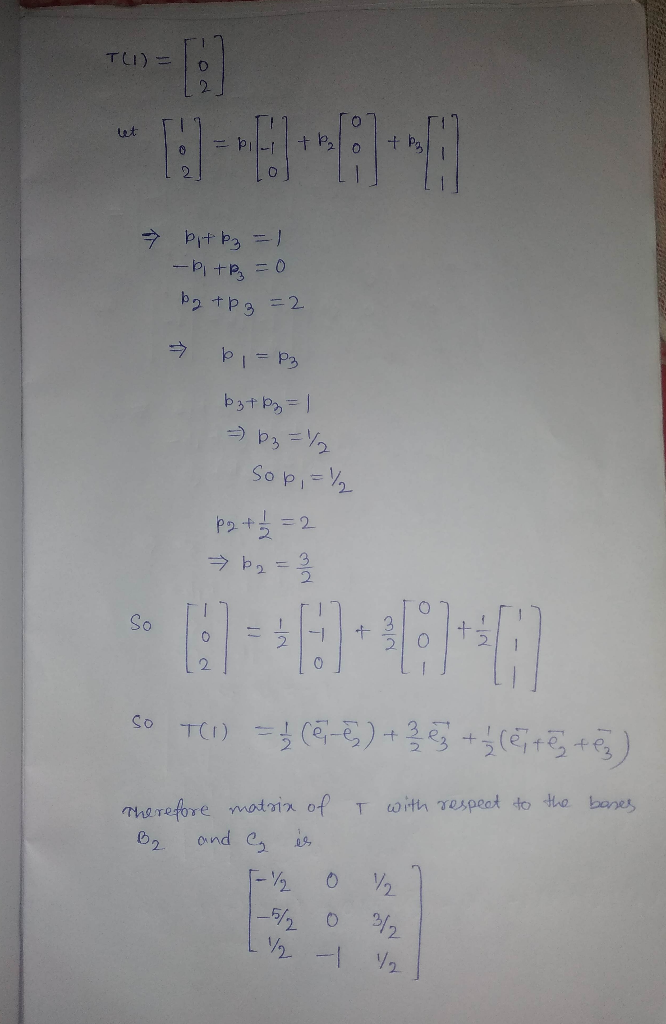#### Earn Coin

Coins can be redeemed for fabulous gifts.

Similar Homework Help Questions
• ### How was the linear transformation of b1 and b2 were applied (L(b1) , L(b2))? NOTE: b1=(1,1)^T , b2=(-1,1)^T Linear Transformations EXAMPLE 4 Let L be a linear transformation mapping R? into itself an...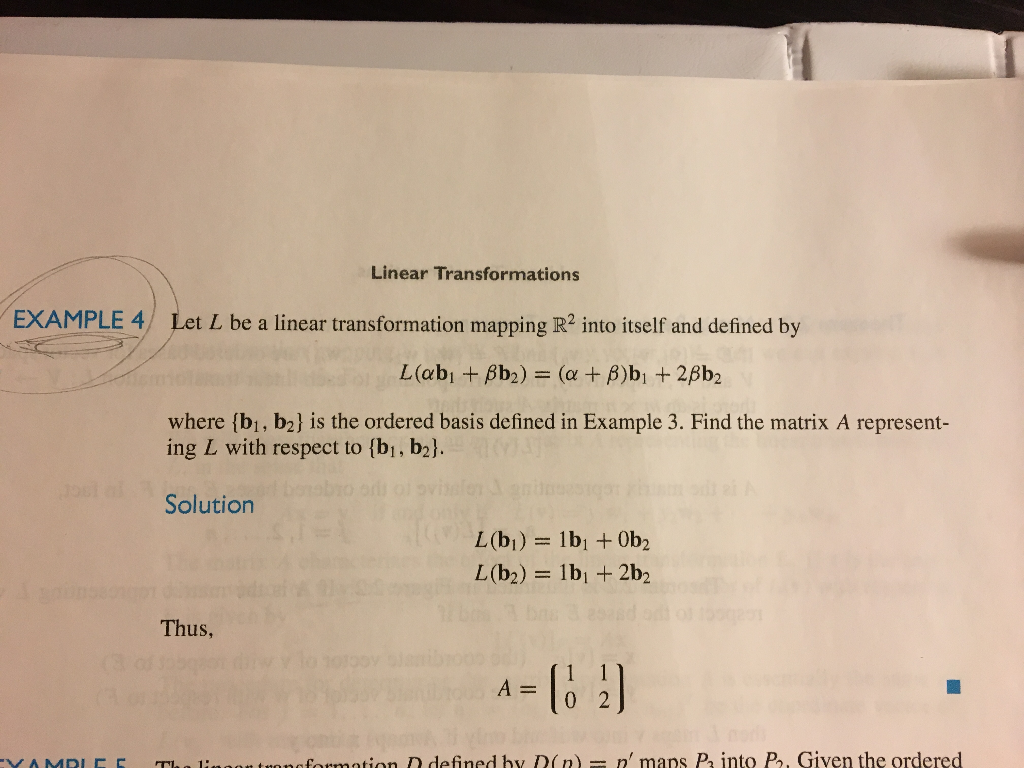How was the linear transformation of b1 and b2 were applied (L(b1) , L(b2))? NOTE: b1=(1,1)^T , b2=(-1,1)^T Linear Transformations EXAMPLE 4 Let L be a linear transformation mapping R? into itself and defined by where (bi, b2] is the ordered basis defined in Example 3. Find the matrix A represent- ing L with respect to [bi, b2l Solution Thus, A0 2 onofosmation D defined by D(n n' maps P into P, Given the ordered Linear Transformations EXAMPLE 4 Let...

• ### Assume that the transition matrix from basis B = {b1, b2, b3} to basis C = {c1, c2, c3} is PC,B = 1/2*[ 0 -1 1 ; -1 1 1 ; 1 0 0 ]. (a) If u = b1 + b2 + 2b3, find [u]C. (b) Calculate PB,C. (c) Suppose...

Assume that the transition matrix from basis B = {b1, b2, b3} to basis C = {c1, c2, c3} is PC,B = 1/2*[ 0 -1 1 ; -1 1 1 ; 1 0 0 ]. (a) If u = b1 + b2 + 2b3, find [u]C. (b) Calculate PB,C. (c) Suppose that c1 = (1, 2, 3), c2 = (1, 2, 0), c3 = (1, 0, 0) and let S be the standard basis for R 3 . (i) Find...

• ### 1) for R2 Given the vectors b1,b2, C1, and cz. B = {b1,b2} is a basis...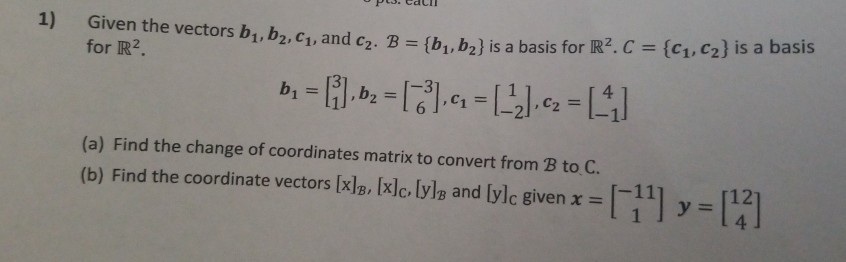1) for R2 Given the vectors b1,b2, C1, and cz. B = {b1,b2} is a basis for R2C = {C1,C2} is a basis b = [i.bz = ,4 = (-2) c2 =  (a) Find the change of coordinates matrix to convert from B to C. (b) Find the coordinate vectors [x]B, [x]c, lyle and [ylc given x =  y = 

• ### Let B = {b1,b2} and C= {(1,62} be bases for R2. Find the change-of-coordinates matrix from...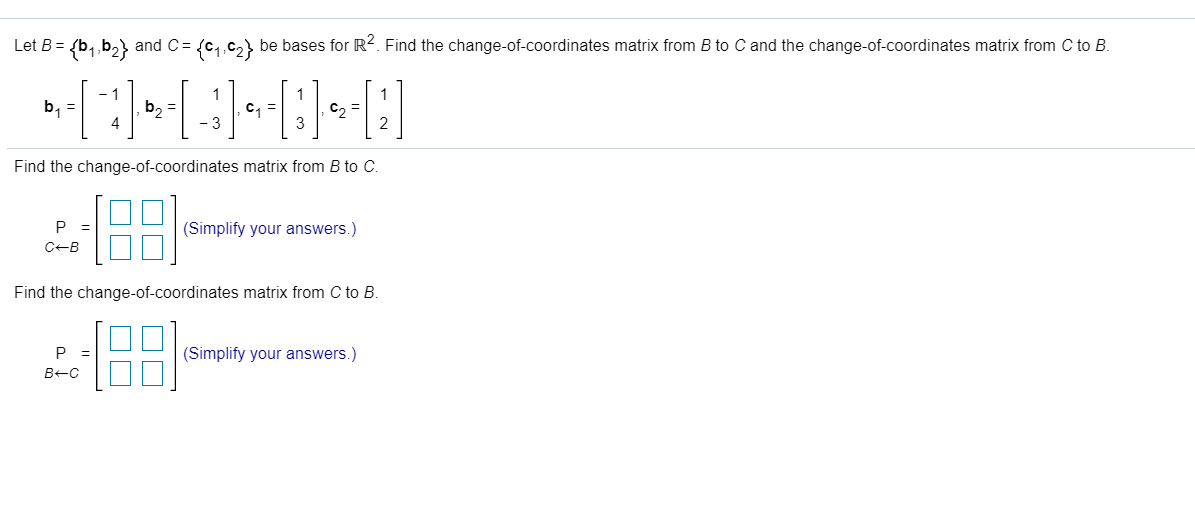Let B = {b1,b2} and C= {(1,62} be bases for R2. Find the change-of-coordinates matrix from B to C and the change-of-coordinates matrix from C to B. - 1 b = b2 = C1 = C = 4 -3 Find the change-of-coordinates matrix from B to C. P = CB (Simplify your answers.) Find the change-of-coordinates matrix from C to B. P B-C [8: (Simplify your answers.)

• ### JI 11 「6 4 Suppose TRF-R2 is defined by TI 1-1":2 il Let S1-G][:)and B2-(AH:)beordered bases...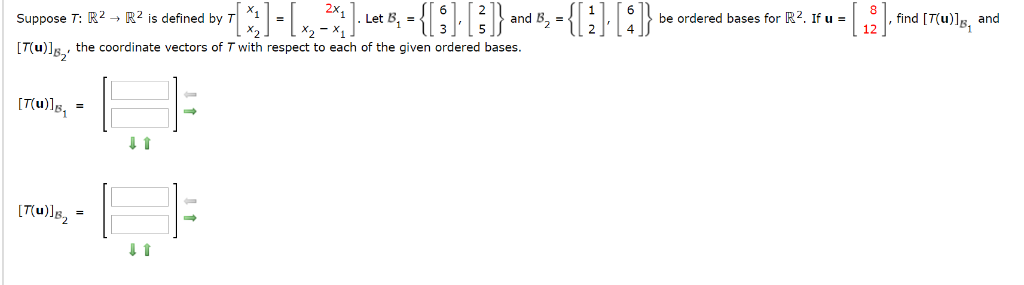JI 11 「6 4 Suppose TRF-R2 is defined by TI 1-1":2 il Let S1-G][:)and B2-(AH:)beordered bases for R2Yu-Cl fin d Mule Suppose T: R2-R2 is defined by T T(u) the coordinate vectors of T with respect to each of the given ordered bases be ordered bases for R2. If u- find [T(u), and and 22X 32 82

• ### (a) Let P(B1∩B2)>0, and A1∪A2⊂B1∩B2. Then show that P(A1|B1).P(A2|B2)=P(A1|B2).P(A2|B1). (b) Let A and B1 be independent;...

(a) Let P(B1∩B2)>0, and A1∪A2⊂B1∩B2. Then show that P(A1|B1).P(A2|B2)=P(A1|B2).P(A2|B1). (b) Let A and B1 be independent; similarly, let A and B2 be independent. Show that in this case, A and B1∪B2 are independent if and only if A and B1∩B2 are independent. (c) Given P(A) = 0.42,P(B) = 0.25, and P(A∩B) = 0.17, find (i)P(A∪B) ; (ii)P(A∩Bc) ; (iii)P(Ac∩Bc) ; (iv)P(Ac|Bc).

• ### Consider a ferrimagnet with two inequivalent sublattices such that the molecular fields B1 and B2 on...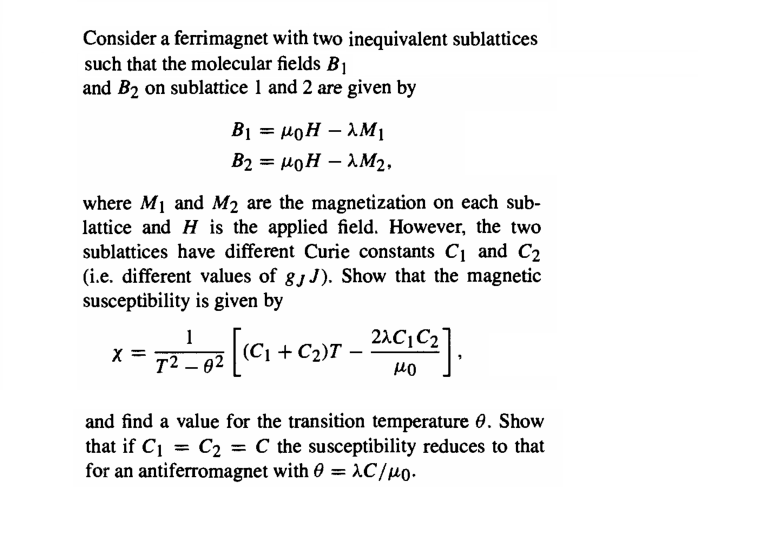Consider a ferrimagnet with two inequivalent sublattices such that the molecular fields B1 and B2 on sublattice 1 and 2 are given by B1 = MOH – AM B2 = MOH - 1M2, where M1 and M2 are the magnetization on each sub- lattice and H is the applied field. However, the two sublattices have different Curie constants C1 and C2 (i.e. different values of 8,1). Show that the magnetic susceptibility is given by x=77_07[1C1 +c-97 – 2002) and find...

• ### Let B = {b1,b2, b3} be a basis for a vector space V. Let T be...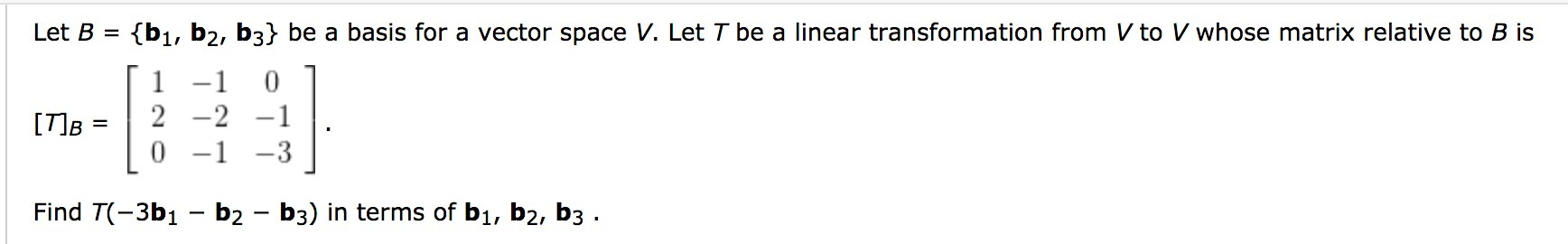Let B = {b1,b2, b3} be a basis for a vector space V. Let T be a linear transformation from V to V whose matrix relative to B is [ 1 -1 0 1 [T]B = 2 -2 -1 . 10 -1 -3 1 Find T(-3b1 – b2 - b3) in terms of bı, b2, b3 .

• ### 0 1 Let S span 1 1 1 0 }, a basis for S. Show that| (a) Let B1 { 1 0 1 1 0 is also a basis for S 0 B2 { 1 (b) Write eac...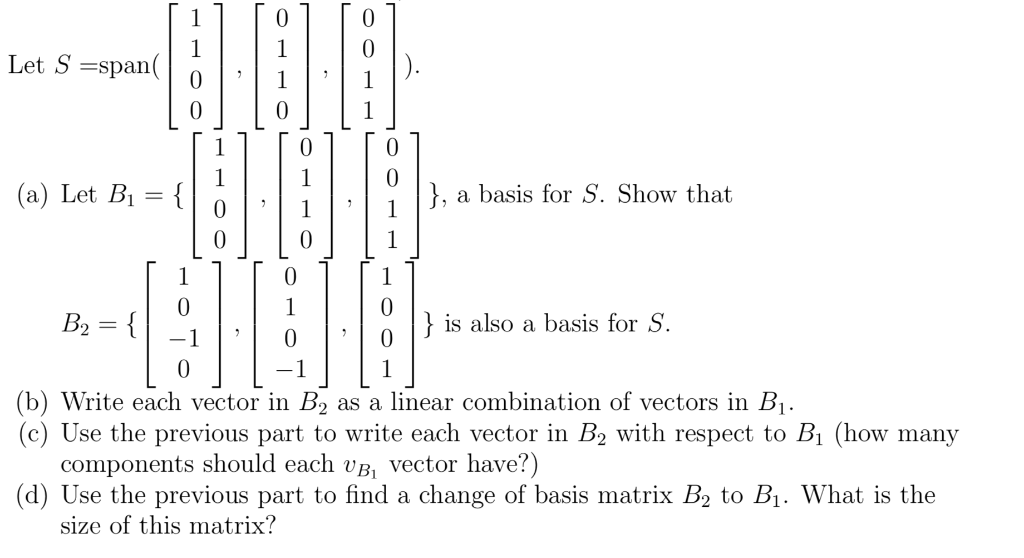0 1 Let S span 1 1 1 0 }, a basis for S. Show that| (a) Let B1 { 1 0 1 1 0 is also a basis for S 0 B2 { 1 (b) Write each vector in B2 (c) Use the previous part to write each vector in B2 with respect to Bi (how many components should each vB, vector have?) (d) Use the previous part to find a change of basis matrix B2 to B1. What...

• ### 1. Consider the following two ordered bases: Bu = {(5), (3) B2 = { 1-2) /1...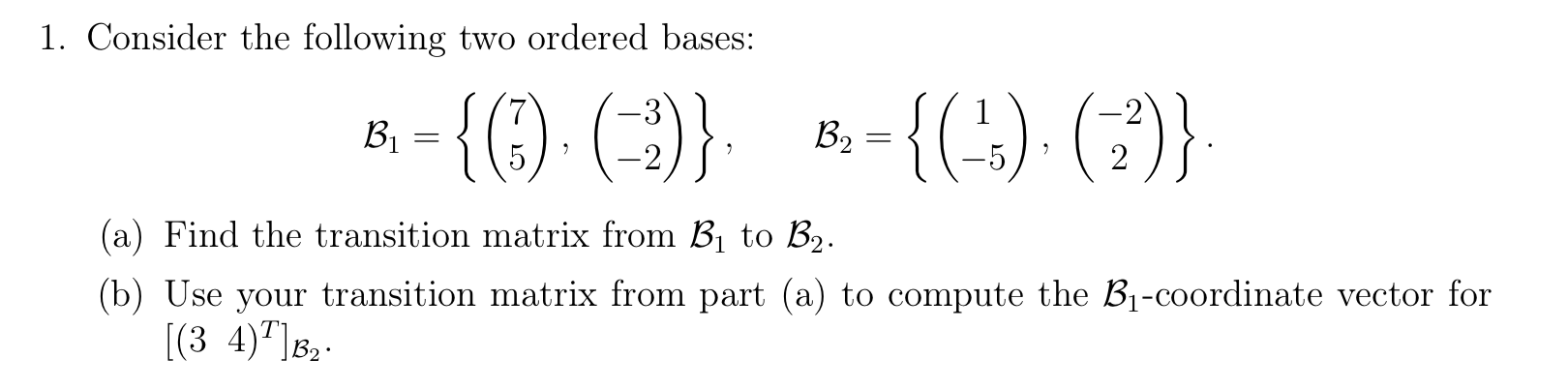1. Consider the following two ordered bases: Bu = {(5), (3) B2 = { 1-2) /1 1-2 ) B = 11-5)' ( 2 ) (a) Find the transition matrix from B1 to B2. (b) Use your transition matrix from part (a) to compute the Bi-coordinate vector for [(3 4)T]12